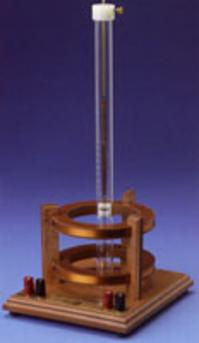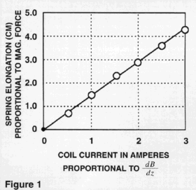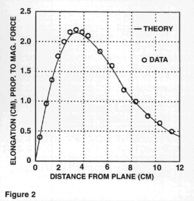## Magnetic Force• Measures Magnetic Moment by Magnetic Force

• Measures dB/dz vs z for a Current Loop

• Resolves Pervasive E&M Misconceptions

## Introduction

MF1-A is a hands-on instrument designed to explore the forces on a permanent magnetic dipole by external magnetic fields. Essentially all introductory texts discuss magnetic forces on dipoles, yet students have not had an opportunity to perform either qualitative or quantitative experiments with this interaction.

The MF1-A uses a small neodymium-iron-boron permanently magnetized disk as a model system for an ideal dipole moment, and a pair of Helmholtz coils to create the external magnetic fields. The various experiments using MF1-A are appropriate for both high school and university students. From TeachSpin's extensive experince demonstrating Magnetic Torque Mt1-A, we are convinced that Magnetic Force experiments will not only surprise (even amaze) most students, but will also serve to clear up on the most pervasive physics misconceptions in E&M. We have developed this affordable apparatus to give students concrete learning experiences with magnetic forces.

## Experiments

Although many qualitative electromagnetism demonstrations are available, Magnetic Force provides hands-on quantitative electromagnetism experiments with which students not only confront misconceptions but also have an opportunity to explore some of the implications of the Biot-Savart Law.

The MF1-A consists of a pair of coils in the Helmholtz configuration and a small magnetized disk mounted so it can rotate in a gimbal which is suspended from a spring. By using Hooke's Law, a measurement of the spring's deflection can be used to infer the magnetic force on the disk. The spring can be calibrated by attaching one-gram steel ball bearings and the coils may be used individually or together in two ways:

• To produce a uniform axial field at the midpoint between the coils

• To produce a field gradient in the same region

Magnetic Force Measurements
There are four main objectives:

1. To demonstrate that there is no net magnetic force on a magnetic dipole in a region of uniform magnetic field. The coils are connected in series with the current circulating in the same direction in both coils.

In the presence of a uniform field of 65 Gauss, the spring does not elongate or compress. This almost always surprises students who expect the dipole to move up or down depending upon the orientation of the dipole with respect to the field. The observation that the dipole only rotates to align itself along the field forces the students to reconsider their preconceived ideas about magnetism.

2. To show that a net magnetic force on a dipole exists only in the presence of a magnetic field gradient, a spatially varying field. For these experiments the coils are connected in series with the currents flowing in opposite directions, creating a field gradient along the axis.

3. To determine the magnitude of the magnetic moment of the dipole by measuring the magnetic force in the field gradient dB/dz. The basic relationship is:

Fm = µ(dB/dz)

The axial magnetic field gradient for N turns of a single coil can be found by differentiating the expression for the axial magnetic field yielding:

dB/dz = (3/2)(µ0IN)(R2z) (R2 + z2)-5/2

Using the physical parameters of the MF1-A coils, students can calculate the field gradient at any axial position. After calibrating the spring, students can measure the magnetic force as a function of field gradient. The magnitude of the magnetic moment can be plotted from the slope of the student data shown in Figure 1. The measurements shown were made at the midpoint of the coils.

4. To examine the axial magnetic field gradient of a single current carrying loop. The magnitude of the field gradient can be determined experimentally from the magnetic force. Students can compare plots of theoretical and experimental values (Figure 2) by taking the derivative of the equation for the axial magnetic field of a current loop, a standard application of the Biot-Savart law.Coils:
168 turns/coil